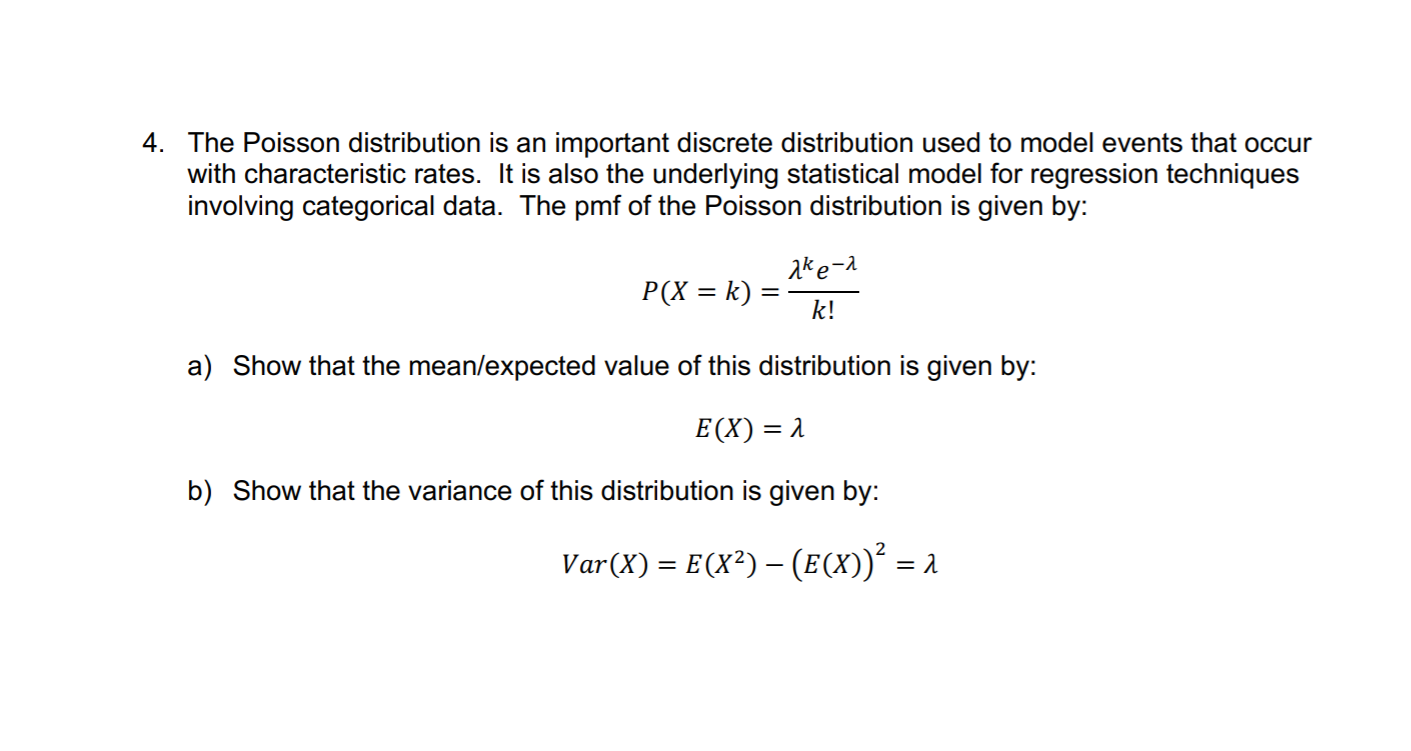# 4. The Poisson distribution is an important discrete distribution used to model events that occurwith characteristic rates. It is also the underlying statistical model for regression techniquesinvolving categorical data. The pmf of the Poisson distribution is given by:ake-2P(X = k)k!a) Show that the mean/expected value of this distribution is given by:E(X) = 1b) Show that the variance of this distribution is given by:Var(X) = E(X²) – (E(X))² = 1

Question
10 viewshelp_outlineImage Transcriptionclose4. The Poisson distribution is an important discrete distribution used to model events that occur with characteristic rates. It is also the underlying statistical model for regression techniques involving categorical data. The pmf of the Poisson distribution is given by: ake-2 P(X = k) k! a) Show that the mean/expected value of this distribution is given by: E(X) = 1 b) Show that the variance of this distribution is given by: Var(X) = E(X²) – (E(X))² = 1 fullscreen
check_circle

star
star
star
star
star
1 Rating
Step 1

a) Expected value:

The expected value is given below:

Step 2

b.

The variance is calculated by th...

### Want to see the full answer?

See Solution

#### Want to see this answer and more?

Solutions are written by subject experts who are available 24/7. Questions are typically answered within 1 hour.*

See Solution
*Response times may vary by subject and question.
Tagged in
MathProbability

### Other